Register for Maths, Science, English, Reasoning Olympiad Exams Register here | Book Free Demo for Live Olympiad Classes here | Check Olympiad Exam Dates here | Buy Practice Papers for IMO, IOM, HEO, IOEL etc here | Login here to participate in all India free mock test on every Saturday

# Money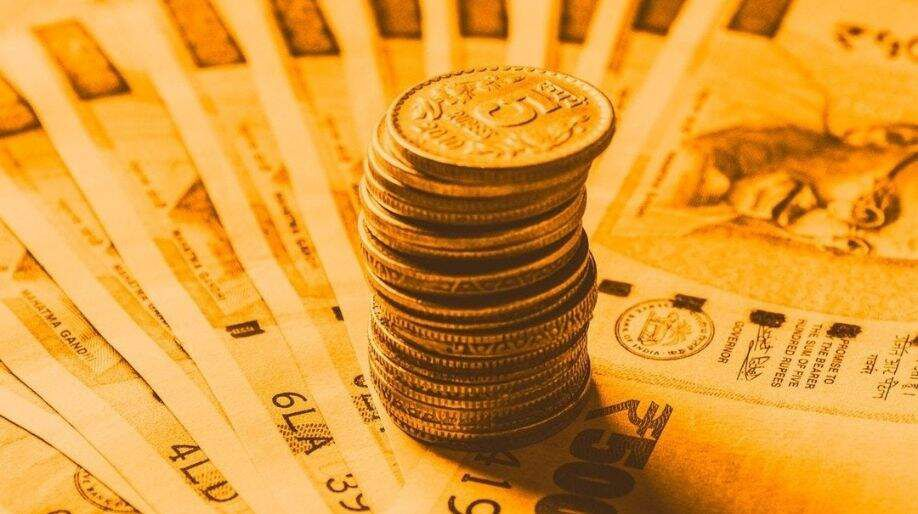• Money is available as (i) Coins and (ii) Notes.
• Coins: The shapes and size of coins changes from time to time. Nowadays one paise to twenty paise coins are not in use. Coins of 50-paise, 1-rupee, 2-rupee and 5-rupee are used mostly.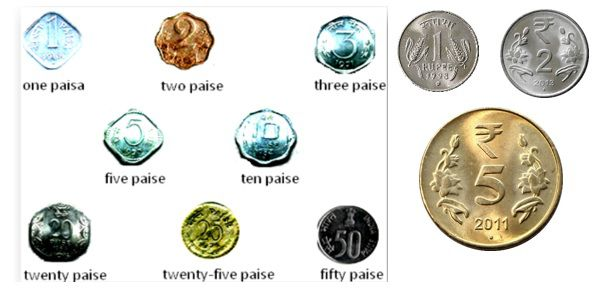• Notes: The designs of currency notes changes from time to time. Both notes and coins of 1-rupee, 2-rupee and 5-rupee denominations are used. One rupee, two-rupee notes are rarely available nowadays. Five-rupee, ten rupees, twenty rupees, fifty rupees, 100 rupees, and 2000 rupees notes are available now.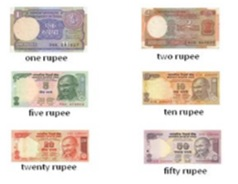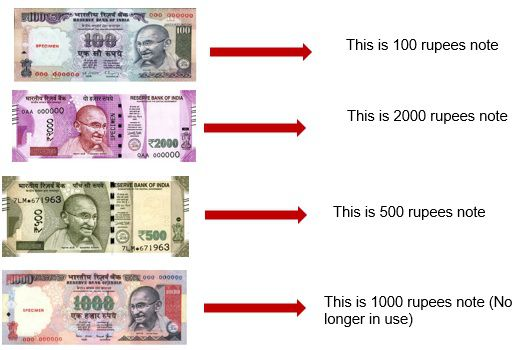EXAMPLE 1: If you want to buy pencils worth Rs.18 and you only have Rs.10 notes and 1 rupee coin, then how will you pay?

SOLUTION: Rs.18 = Rs.10 + 1 + 1 + 1 + 1 + 1 + 1 + 1 + 1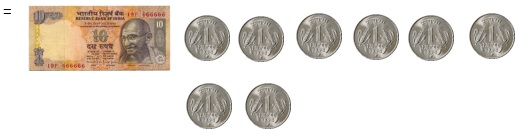EXAMPLE 2: How many notes and coins do you need to give 135 rupees?

SOLUTION: You give me 1 hundred rupees note three 10-rupees notes and 5 coins of one rupee.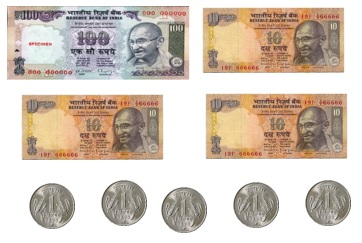EXAMPLE 3: Draw the10-rupee notes and 1-rupee coins you will give for these things.

SOLUTION: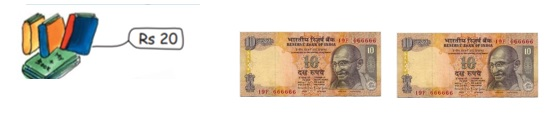# Practice these questions

Q1) Draw the10-rupees notes and 1-rupee coins you will give for these things.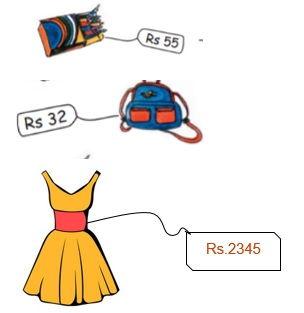Q2) How much money do the notes and the coins make?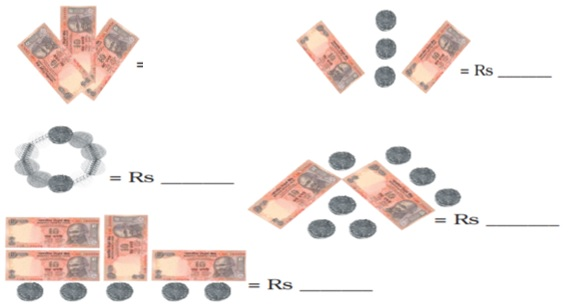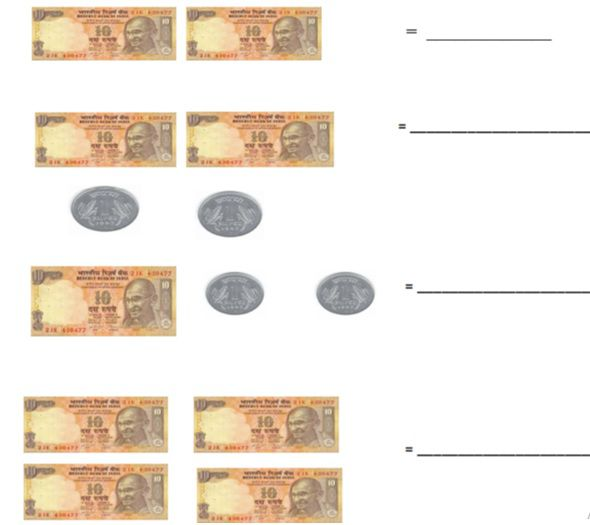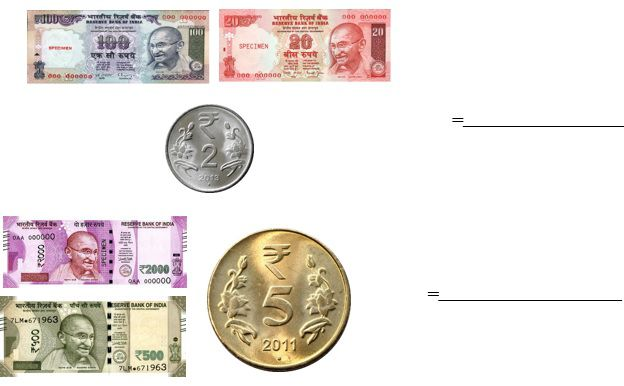## Quiz for Money

 Q.1 Rajesh bought a cake of Rs. 25, a coke of Rs. 13 and a burger of Rs. 30. He gave a note of Rs. 100 to the shopkeeper. How much change did he got? a) Rs. 32 b) Rs. 30 c) Rs. 28 d) Rs. 26
 Q.2 Sunita bought 12 pencils of Rs. 5 each. How much did she pay? a) Rs. 45 b) Rs. 60 c) Rs. 75 d) Rs.48
 Q.3 Ram has a loan of Rs. 1000 from the Bank of India with an interest rate of 5% . How much did he pay as interest? a) Rs. 50 b) Rs. 200 c) Rs. 100 d) Rs. 250
 Q.4 If 1\$ = 67.56, then how much money in Indian currency do I have if I have 10\$? a) Rs. 6.756 b) Rs. 67.56 c) Rs. 675.6 d) Rs. 6756
 Q.5 Raj gave a 50 rupees note to the shopkeeper. How much money did he get back?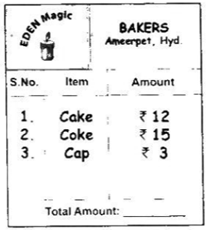a) Rs. 20 b) Rs. 15 c) Rs. 30 d) Rs. 25
 Q.6 Which of the following is the cheapest? a)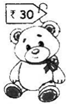b)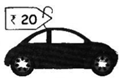c)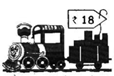d)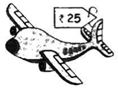Q.7 If each apple costs Rs. 7, then how much both the baskets cost?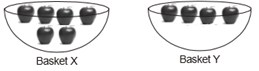a) Rs. 50 b) Rs. 60 c) Rs. 70 d) Rs. 80
 Q.8 Which of the following is the most expensive item? a)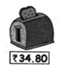b)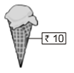c)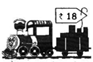d)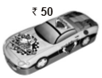Q.9 Kriti wants to buy treats for her 5 dogs. At the pet store, she sees the boxes of treats as shown. She buys the boxes so each of her dogs can receive an equal number of treats with one treat left over. How many treats should she buy? a)b)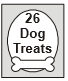c)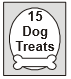d)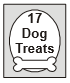Q.10 If one chit is equal to Rs. 100, then how much did the shopkeeper earned that day?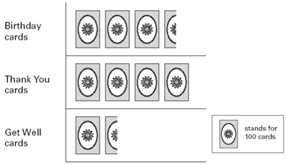a) Rs. 450 b) Rs. 900 c) Rs. 650 d) Rs. 750